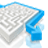# SimplificationQuantitative Aptitude Questions and Answers section on “Simplification” with solution and explanation for competitive examinations such as CAT, MBA, SSC, Bank PO, Bank Clerical and other examinations.

# Aptitude Question ID : 94830

The value of is : [A] [B] [C] [D] Show Answer Hence option [D] is right answer.

# Aptitude Question ID : 94821

On simplification, the expression is equal to : [A] [B] [C] [D] Show Answer Hence option [D] is right answer.

# Aptitude Question ID : 94817

The value of is : [A]0 [B]1 [C]1 – a [D]a Show Answer 0 Hence option [A] is right answer.

# Aptitude Question ID : 94813

The value of is : [A] [B] [C] [D] Show Answer Hence option [B] is right answer.

# Aptitude Question ID : 94807

Find out the value of [A]1 [B]2 [C]3 [D]4 Show Answer 1 Hence option [A] is right answer.